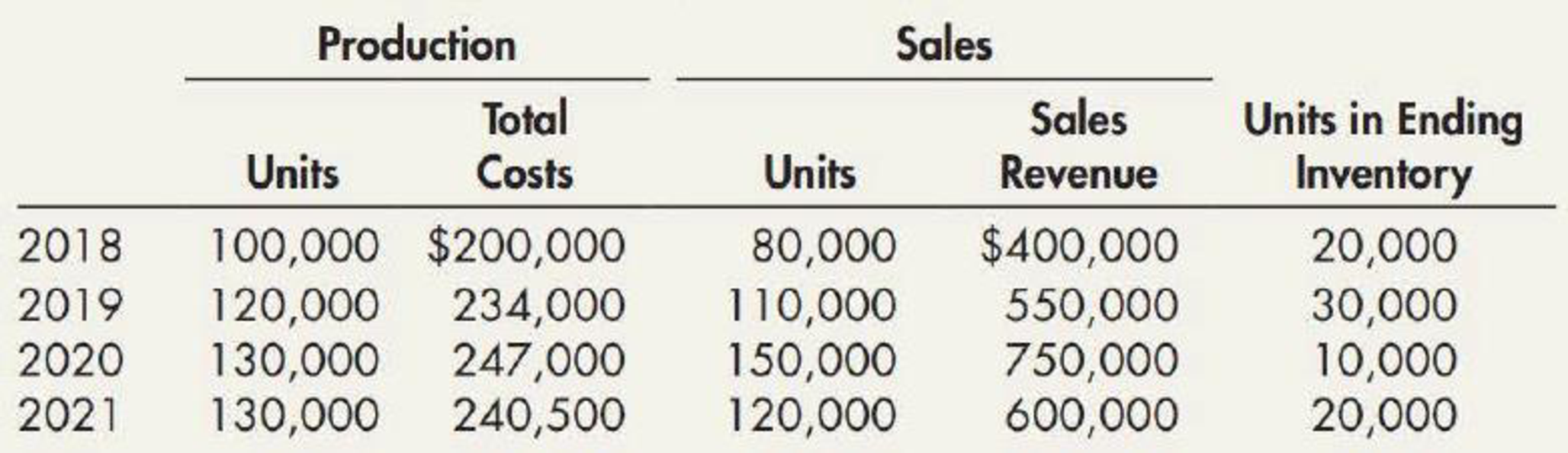Chapter 7, Problem 15E### Intermediate Accounting: Reporting...

3rd Edition
James M. Wahlen + 2 others
ISBN: 9781337788281

#### Solutions

Chapter
Section### Intermediate Accounting: Reporting...

3rd Edition
James M. Wahlen + 2 others
ISBN: 9781337788281
Textbook Problem
12 views

# Habicht Company was formed in 2018 to produce a single product. The production and sales for the next 4 years were as follows:Required: 1. Determine the gross profit for each year under each of the following periodic inventory methods: a. FIFO b. LIFO c. Average cost (Round unit costs to 3 decimal places.) 2. Next Level Explain whether the company’s return on assets (net income divided by average total assets) would be higher under FIFO or LIFO.

1.

To determine

Ascertain the gross profit under the periodic inventory system for each year as per the inventory cashflow methods.

Explanation

First-in-First-Out (FIFO): In this method, items purchased initially are sold first. So, the value of the ending inventory consists the recent cost for the remaining unsold items.

Last-in-First-Out (LIFO): In this method, items purchased recently are sold first. So, the value of the ending inventory consists the initial cost for the remaining unsold items.

1. a)      Calculate the gross profit for the four years under FIFO method:
 Particulars 2018 2019 2020 2021 Amount ($) Amount ($) Amount ($) Amount ($) Net sales $400,000$550,000 $750,000$600,000 Cost of goods sold Beginning inventory $0$ 40,000 $58,500$ 19,000 Add: Production $200,000$ 234,000 $247,000$ 240,500 Goods available for sale $200,000$ 274,000 $305,500$ 259,500 Less: Ending inventory (1) $40,000 (2)$ 58,500 (3) $19,000 (4)$ 37,000 Cost of goods sold $160,000$ 215,500 $286,500$ 222,500 Gross Margin $240,000$ 334,500 $463,500$ 377,500

Table (1)

Working Note (1):

Ending inventory for 2018 is $40,000(20,000 units×$2.00).

Working Note (2):

Ending inventory for 2019 is $58,500(30,000 units×$1.95).

Working Note (3):

Ending inventory for 2020 is $19,000(10,000 units×$1.90).

Working Note (4):

Ending inventory for 2021 is $37,000(20,000 units×$1.85).

1. b)     Calculate the gross profit for the four years under LIFO method:
 Particulars 2018 2019 2020 2021 Amount ($) Amount ($) Amount ($) Amount ($) Net sales $400,000$550,000 $750,000$600,000 Cost of goods sold Beginning inventory $0$ 40,000 $59,500$ 20,000 Add: Production $200,000$ 234,000 $247,000$ 240,500 Goods available for sale $200,000$ 274,000 $306,500$ 260,500 Less: Ending inventory (5) $40,000 (6)$ 59,500 (7) $20,000 (8)$ 38,500 Cost of goods sold $160,000$ 214,500 $286,500$ 222,000 Gross Margin $240,000$ 335,500 $463,500$ 378,000

Table (2)

Working Note (5):

Ending inventory for 2018 is $40,000(20,000 units×$2

2.

To determine

Identify would return on asset would be greater under FIFO and LIFO.

### Still sussing out bartleby?

Check out a sample textbook solution.

See a sample solution

#### The Solution to Your Study Problems

Bartleby provides explanations to thousands of textbook problems written by our experts, many with advanced degrees!

Get Started

#### What are control limits, and how are they set?

Managerial Accounting: The Cornerstone of Business Decision-Making

#### How did the customer orientation evolve?

Foundations of Business (MindTap Course List)

#### What does the invisible hand of the marketplace do?

Principles of Microeconomics (MindTap Course List)

#### UNEVEN CASH FLOW STREAM a. Find the present values of the following cash flow streams at an 8% discount rate. b...

Fundamentals of Financial Management, Concise Edition (with Thomson ONE - Business School Edition, 1 term (6 months) Printed Access Card) (MindTap Course List)